# Slicing, Indexing, Manipulating and Cleaning Pandas Dataframe

• Difficulty Level : Expert
• Last Updated : 05 Sep, 2020

With the help of Pandas, we can perform many functions on data set like Slicing, Indexing, Manipulating, and Cleaning Data frame.

Case 1: Slicing Pandas Data frame using DataFrame.iloc[]

Example 1: Slicing Rows

## Python3

 `# importing pandas library``import` `pandas as pd`` ` `# Initializing the nested list with Data set``player_list ``=` `[[``'M.S.Dhoni'``, ``36``, ``75``, ``5428000``], ``               ``[``'A.B.D Villers'``, ``38``, ``74``, ``3428000``], ``               ``[``'V.Kholi'``, ``31``, ``70``, ``8428000``],``               ``[``'S.Smith'``, ``34``, ``80``, ``4428000``], ``               ``[``'C.Gayle'``, ``40``, ``100``, ``4528000``],``               ``[``'J.Root'``, ``33``, ``72``, ``7028000``],``               ``[``'K.Peterson'``, ``42``, ``85``, ``2528000``]]`` ` `# creating a pandas dataframe``df ``=` `pd.DataFrame(player_list, columns``=``[``'Name'``, ``'Age'``, ``'Weight'``, ``'Salary'``])`` ` `# data frame before slicing``df`

Output: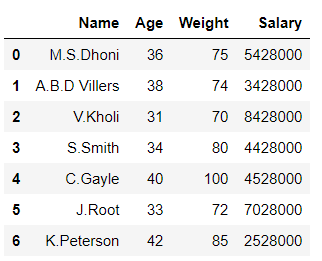## Python3

 `# Slicing rows in data frame``df1 ``=` `df.iloc[``0``:``4``]`` ` `# data frame after slicing``df1`

Output: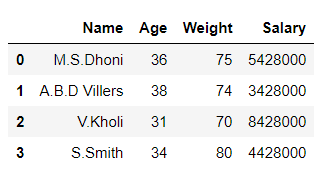In the above example, we sliced the rows from the data frame.

Example 2: Slicing Columns

## Python3

 `# importing pandas library``import` `pandas as pd`` ` `# Initializing the nested list with Data set``player_list ``=` `[[``'M.S.Dhoni'``, ``36``, ``75``, ``5428000``],``               ``[``'A.B.D Villers'``, ``38``, ``74``, ``3428000``],``               ``[``'V.Kholi'``, ``31``, ``70``, ``8428000``],``               ``[``'S.Smith'``, ``34``, ``80``, ``4428000``],``               ``[``'C.Gayle'``, ``40``, ``100``, ``4528000``],``               ``[``'J.Root'``, ``33``, ``72``, ``7028000``], ``               ``[``'K.Peterson'``, ``42``, ``85``, ``2528000``]]`` ` `# creating a pandas dataframe``df ``=` `pd.DataFrame(player_list, columns``=``[``'Name'``, ``'Age'``, ``'Weight'``, ``'Salary'``])`` ` `# data frame before slicing``df`

Output:## Python3

 `# Slicing columnss in data frame``df1 ``=` `df.iloc[:,``0``:``2``]`` ` `# data frame after slicing``df1`

Output: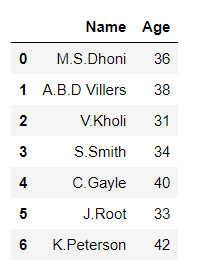In the above example, we sliced the columns from the data frame.

Case 2: Indexing Pandas Data frame

## Python3

 `# importing pandas library``import` `pandas as pd`` ` `# Initializing the nested list with Data set``player_list ``=` `[[``'M.S.Dhoni'``, ``36``, ``75``, ``5428000``], ``               ``[``'A.B.D Villers'``, ``38``, ``74``, ``3428000``],``               ``[``'V.Kholi'``, ``31``, ``70``, ``8428000``],``               ``[``'S.Smith'``, ``34``, ``80``, ``4428000``], ``               ``[``'C.Gayle'``, ``40``, ``100``, ``4528000``],``               ``[``'J.Root'``, ``33``, ``72``, ``7028000``], ``               ``[``'K.Peterson'``, ``42``, ``85``, ``2528000``]]`` ` `# creating a pandas dataframe and indexing it using Aplhabets``df ``=` `pd.DataFrame(player_list, columns``=``[``'Name'``, ``'Age'``, ``'Weight'``, ``'Salary'``],``                  ``index``=``[``'A'``, ``'B'``, ``'C'``, ``'D'``, ``'E'``, ``'F'``, ``'G'``])`` ` ` ` `# Displaying data frame``df`

Output: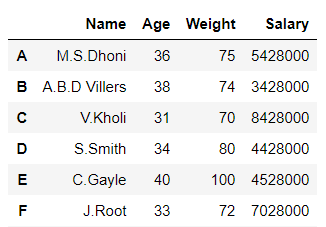In the above example, we do indexing of the data frame.

Case 3: Manipulating Pandas Data frame

Manipulation of the data frame can be done in multiple ways like applying functions, changing a data type of columns, splitting, adding rows and columns to a data frame, etc.

Example 1: Applying lambda function to a column using Dataframe.assign()

## Python3

 `# importing pandas library``import` `pandas as pd`` ` `# creating and initializing a list``values ``=` `[[``'Rohan'``, ``455``], [``'Elvish'``, ``250``], [``'Deepak'``, ``495``],``          ``[``'Sai'``, ``400``], [``'Radha'``, ``350``], [``'Vansh'``, ``450``]]`` ` `# creating a pandas dataframe``df ``=` `pd.DataFrame(values, columns``=``[``'Name'``, ``'Univ_Marks'``])`` ` `# Applying lambda function to find percentage of``# 'Univ_Marks' column using df.assign()``df ``=` `df.assign(Percentage``=``lambda` `x: (x[``'Univ_Marks'``] ``/` `500` `*` `100``))`` ` `# displaying the data frame``df`

Output: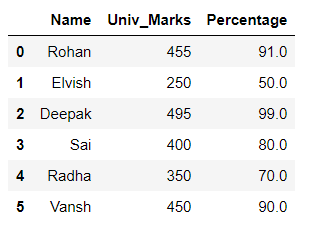In the above example, the lambda function is applied to the ‘Univ_Marks’ column and a new column ‘Percentage’ is formed with the help of it.

Example 2: Sorting the Data frame in Ascending order

## Python3

 `# importing pandas library``import` `pandas as pd`` ` `# Initializing the nested list with Data set``player_list ``=` `[[``'M.S.Dhoni'``, ``36``, ``75``, ``5428000``],``               ``[``'A.B.D Villers'``, ``38``, ``74``, ``3428000``],``               ``[``'V.Kholi'``, ``31``, ``70``, ``8428000``],``               ``[``'S.Smith'``, ``34``, ``80``, ``4428000``], ``               ``[``'C.Gayle'``, ``40``, ``100``, ``4528000``],``               ``[``'J.Root'``, ``33``, ``72``, ``7028000``],``               ``[``'K.Peterson'``, ``42``, ``85``, ``2528000``]]`` ` `# creating a pandas dataframe``df ``=` `pd.DataFrame(player_list, columns``=``[``'Name'``, ``'Age'``, ``'Weight'``, ``'Salary'``])`` ` `# Sorting by column 'Weight'``df.sort_values(by``=``[``'Weight'``])`

Output: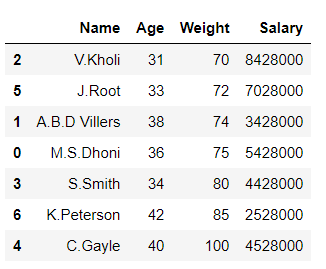In the above example, we sort the data frame by column ‘Weight”.

Case 4: Cleaning Pandas Data frame

## Python3

 `# importing pandas and Numpy libraries``import` `pandas as pd``import` `numpy as np`` ` `# Initializing the nested list with Data set``player_list ``=` `[[``'M.S.Dhoni'``, ``36``, ``75``, ``5428000``],``               ``[``'A.B.D Villers'``, np.nan, ``74``, np.nan],``               ``[``'V.Kholi'``, ``31``, ``70``, ``8428000``],``               ``[``'S.Smith'``, ``34``, ``80``, ``4428000``],``               ``[``'C.Gayle'``, np.nan, ``100``, np.nan],``               ``[np.nan, ``33``, np.nan, ``7028000``], ``               ``[``'K.Peterson'``, ``42``, ``85``, ``2528000``]]`` ` `# creating a pandas dataframe``df ``=` `pd.DataFrame(player_list, columns``=``[``'Name'``, ``'Age'``, ``'Weight'``, ``'Salary'``])`` ` `df`

Output:## Python3

 `# Checking for missing values``df.isnull().``sum``()`

Output: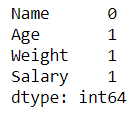## Python3

 `# dropping or cleaning the missing data ``df``=` `df.dropna() ``df`

Output: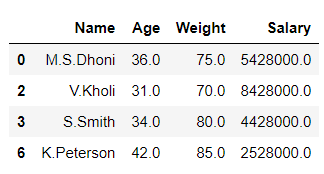In the above example, we clean all the missing values from the data set.

My Personal Notes arrow_drop_up